# Looking at Alternatives with Data TablesYou can look to see a range of possible values for your formulas. Data tables provide a shortcut by calculating all of the values in one operation. A data table is a range of cells that shows the results of substituting different values in one or more formulas. For example, you can compare loan payments for different interest rates. There are two types of data tables: one-input and two-input. With a one-input table , you enter different values for one variable and see the effect on one or more formulas. With a two-input table , you enter values for two variables and see the effect on one formula.

#### Create a One-Input Data Table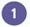Enter the formula you want to use.

If the input values are listed down a column, specify the new formula in a blank cell to the right of an existing formula in the top row of the table. If the input values are listed across a row, enter the new formula in a blank cell below an existing formula in the first column of the table.Select the data table, including the column or row that contains the new formula.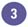Click the Data menu, and then click Table.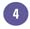Enter the input cell.

If the input values are in a column, enter the reference for the input cell in the Column Input Cell box. If the input values are in a row, enter the reference for the input cell in the Row Input Cell box.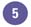Click OK.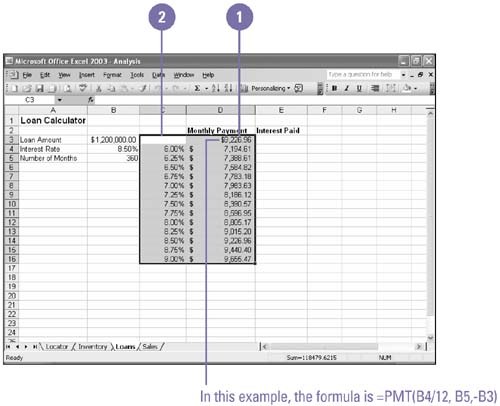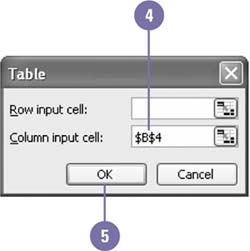Show Me Microsoft Office Excel 2003
ISBN: 0789730057
EAN: 2147483647
Year: 2002
Pages: 291